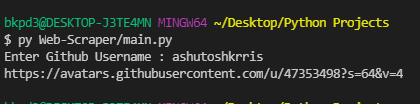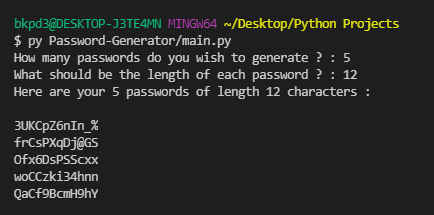# 5 Quick Python Projects

ProgrammingIn this blog, we'll be building five different Python projects. These projects will be a little bit above the beginner's level, so we can consider them as Intermediate Python Projects. So, we are going to build the below mentioned five projects :

1. Web Scraping Program
2. Renaming Bulk Files
3. Getting Weather Information
5. QR Codes with Python

# Web Scraping Program

In this project, we'll be using Web Scraping to get the image link of the profile image of a particular Github user. So, what our program is going to do, it's going to ask for a Github username and then it will return the image link of that account.Before we start we need to install two external Python libraries - requests and bs4

``pip install requests bs4``

The above command will install these two libraries and now we are ready for coding.

So, the first thing we need to do is to import these libraries in our code :

``````import requests
from bs4 import BeautifulSoup as bs``````

Now, let's understand how we are going to proceed. First, we are going to fetch the user's profile on Github using requests and pass all the HTML content of the page to the BeautifulSoup to parse using an HTML parser. Now, we need to understand one thing. To fetch the user's image, we need to visit its profile and inspect the HTML content first in order to visualize how we are going to extract the image. SO, after inspecting, we have this HTML content that is used for the profile image (as of now):

``<img style="height:auto;" alt="" width="260" height="260" class="avatar avatar-user width-full border color-bg-primary" src="https://avatars.githubusercontent.com/u/47353498?v=4">``

In this HTML content, we need to find a unique attribute using which we can fetch the src of the img tag. We can use the class avatar avatar-user for this, and this way we can get the image link. Now let's jump to the code :

``````def get_profile_image(username) -> str:
res = requests.get(url)
soup = bs(res.content, 'html.parser')
profile_image = soup.select('img.avatar.avatar-user')['src']
return profile_image``````

In the above code, we are first making a GET request on the user's profile and then using the select method in soup to get the image tag with class avatar AND avatar-user. This will return us a list of all the image tags with this class. We need to get the first element of the list and get its src.

So, our entire code is now :

``````import requests
from bs4 import BeautifulSoup as bs

res = requests.get(url)
soup = bs(res.content, 'html.parser')
profile_image = soup.select('img.avatar.avatar-user')['src']
return profile_image

if __name__ == "__main__":

Note : This code cannot be used later since the HTML content may change in future.

# Renaming Bulk Files

In this project, we are going to write a Python program to rename all files within a folder. First, let's create a folder named demo and put three text files named file1.txt, file2.txt and file3.txt .  Let's start coding this.

First we need to import the os library.

``import os``

Let's create a function to function called rename_files which takes an argument path.

``````def rename_files(path):
i = 0
for file in os.listdir(path):
new_name = f"Hello{i}.txt"
src = path+file
new_name = path+new_name
os.rename(src, new_name)
i += 1
``````

In this method, we will iterate over all the files within a given path and use the rename method in os to rename it with the new name given by f"Hello{i}.txt". We are using i as an increment variable.

So, the entire code is now :

``````import os

def rename_files(path):
i = 0
for file in os.listdir(path):
new_name = f"Hello{i}.txt"
src = path+file
new_name = path+new_name
os.rename(src, new_name)
i += 1

if __name__ == "__main__":
rename_files("Renaming-Bulk-Files/demo/")
``````

After running the program, all the files will be renamed as Hello1.txt, Hello2.txt and Hello3.txt respectively.

# Getting Weather Information

In this project, we are building a weather program that will tell current weather situation in a city or town or any location. So, for us to do this, we will be using Open Weather Maps API. Just signup for free and get an API Key. We'll require requests library for this too, and we have already installed that.

Let's start coding.

First we need to import the libraries :

``````import requests
from pprint import pprint
``````

When we make request on the API, it responds with JSON data which is not easy to read, So, the pprint (pretty print) library will make JSON data readable.

Also, set the below two global variables :

``````API_KEY = '1e121683f8158a495a3414b71abcdef'
BASE_URL = 'https://api.openweathermap.org/data/2.5/weather'``````

You can get the API Key and Base URL from the above mentioned site.
Now, let's create a function to get the weather data.

``````def get_weather_condition(city):
query_params = {
'appid': API_KEY,
'q': city
}
res = requests.get(BASE_URL, params=query_params).json()
pprint(res)``````

In the above function, we are first creating a query params dictionary having two key-value pairs called appid and q where appid is the API Key and q is the city which we want to query. Then we are making a GET request to the Base URL with query_params as params and thus our resultant URL will become:

``https://api.openweathermap.org/data/2.5/weather?appid=API_KEY&q=city``

We will get a JSON response and hence we are using the pprint library to make it readable. Our response will look like this :

``````{'base': 'stations',
'clouds': {'all': 75},
'cod': 200,
'coord': {'lat': 24.7833, 'lon': 85},
'dt': 1622302757,
'id': 1271439,
'main': {'feels_like': 303.06,
'humidity': 83,
'pressure': 1004,
'temp': 300.1,
'temp_max': 300.1,
'temp_min': 300.1},
'name': 'Gaya',
'sys': {'country': 'IN',
'id': 9115,
'sunrise': 1622244683,
'sunset': 1622293424,
'type': 1},
'timezone': 19800,
'visibility': 2000,
'weather': [{'description': 'broken clouds',
'icon': '04n',
'id': 803,
'main': 'Clouds'}],
'wind': {'deg': 0, 'speed': 0}}``````

We can see that it responds with the current weather conditions of the city that we asked the data for. We can use this program anywhere like in a GUI application or a web application.

So, our complete program looks like :

``````import requests
from pprint import pprint

API_KEY = '1e121683f8158a495a3414b7abcdef'
BASE_URL = 'https://api.openweathermap.org/data/2.5/weather'

def get_weather_condition(city):
query_params = {
'appid': API_KEY,
'q': city
}
res = requests.get(BASE_URL, params=query_params).json()
pprint(res)

if __name__ == "__main__":
city = input("Enter city name : ")
get_weather_condition(city)
``````

In this project, we are going to build a Password Generator that will generate some random passwords of some length.In this project, we'll be making use of Python's random module and string module. So, first we need to import them as :

``````from random import choice
import string``````

Since, we'll be using only the choice method from random module, we have imported it explicitly.

Let's declare some global variables that we are going to use :

``````UPPERCASE = string.ascii_uppercase
LOWECASE = string.ascii_lowercase
NUMBER = string.digits
SYMBOLS = ['@', '#', '\$', '%', '&', '_']
DATA = list(UPPERCASE) + list(LOWECASE) + list(NUMBER) + SYMBOLS``````

Using the string library, we are creating three variables that contains Uppercase letters, Lowercase letters and Digits respectively. Apart from that we are having Symbols list that has few symbols. Using all of these four, we are creating a list called DATA that we'll be making use of.

Now, let's create a method called generate_random_password that takes two arguments : length and number.

``````def generate_random_password(length, number):
for _ in range(number):
password = ''.join(choice(DATA) for _ in range(length))

Now we are creating a password using the choice method that generates a random string of length length using the DATA list. We are repeating the same for the number number of times.

So,our complete program now looks like :

``````from random import choice
import string

UPPERCASE = string.ascii_uppercase
LOWECASE = string.ascii_lowercase
NUMBER = string.digits
SYMBOLS = ['@', '#', '\$', '%', '&', '_']
DATA = list(UPPERCASE) + list(LOWECASE) + list(NUMBER) + SYMBOLS

for _ in range(number):
password = ''.join(choice(DATA) for _ in range(length))

if __name__ == "__main__":
number = int(input("How many passwords do you wish to generate ? : "))
length = int(input("What should be the length of each password ? : "))
``````

# QR Codes in Python

In this project, we'll be talking about encoding and decoding QR Codes in Python.

Let's start by first encoding a QR Code and by encoding we mean, adding some data to a QR Code. For this we have several libraries in Python but we'll be making use of qrcode library. Also, this library requires Pillow internally. So, first we need to install these packages :

``pip install qrcode Pillow``

Now we can use the qrcode module to create a QR Code.

``````def encode_qr(data):
qr = qrcode.QRCode(version=1, box_size=10, border=5)
qr.make(fit=True)
img = qr.make_image(fill_color='black', back_color='white')
img.save('QR-Codes-in-Python/qrcode.png')``````

In the above function, we are first defining the version, box_size and border of the QR code and then adding the data into it. We want a standard QR Code and hence set the colors to black and white and then we are saving the QR code as a PNG image file. Learn more about QR Codes here.Now, let's decode the same QR code. For this, we require another library called pyzbar. So let's install this.

``pip install pyzbar``

Then we can import libraries and create a decode_qr function as :

``````from pyzbar.pyzbar import decode
from PIL import Image

def decode_qr(image):
img = Image.open(image)
result = decode(img)
print(result)``````

This is a simple function which will decode the QR code immediately.

So our complete code becomes :

``````import qrcode
from pyzbar.pyzbar import decode
from PIL import Image

def encode_qr(data):
qr = qrcode.QRCode(version=1, box_size=10, border=5)
qr.make(fit=True)
img = qr.make_image(fill_color='black', back_color='white')
img.save('QR-Codes-in-Python/qrcode.png')

def decode_qr(image):
img = Image.open(image)
result = decode(img)
print(result)

if __name__ == "__main__":